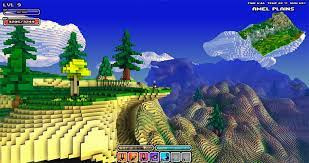# Cube World Voxel Terrain Generation

so, again. I’m making a voxel game similar to cube world, and I wanted to know how to generate mountain and mountain overhangs like cubeworld.

here is the script

``````local seed = math.random(1,1)
local mapSize = 500

local coveredBlocks = {}

for xAxis = 1, mapSize do
for zAxis = 1, mapSize do
local noise = math.noise(seed, xAxis/60, zAxis/60)*8
local block = script.Parent.Block:Clone()
local yPos = noise
if yPos <= -3 then
block.BrickColor = BrickColor.new("Shamrock")
end
if yPos >= 2 then
block.BrickColor = BrickColor.new("Dark stone grey")
end
block.Position = Vector3.new(xAxis*4, math.floor(noise)*3, zAxis*4)
block.Parent = workspace.RandomGeneratedMap

if math.random(1, 5000) == 1 then

if xAxis <= 1 or zAxis <= 1 or xAxis >= mapSize-1 or zAxis >= mapSize-1 then continue end

noise = math.floor(noise)

local flat = true
for x = -1, 1 do
if coveredBlocks[(xAxis+x)] == nil then coveredBlocks[(xAxis+x)] = {} end
for z = -1, 1 do
if coveredBlocks[(xAxis+x)][(zAxis+z)] or noise ~= math.floor(math.noise(seed, (xAxis+x)/60, (zAxis+z)/60)*8) then
flat = false
break
end
end
end

if flat then
local structure = script.Parent["Life Shrine"]:Clone()
structure.Parent = workspace.RandomGeneratedMap.Structures
structure:MoveTo(block.Position)

for x = -1, 1 do
if coveredBlocks[(xAxis+x)] == nil then coveredBlocks[(xAxis+x)] = {} end

for z = -1, 1 do
coveredBlocks[(xAxis+x)][(zAxis+z)] = true
end
end
end
end
end
end
``````

of course, you don’t need to change the entire script and send it to me, I just want to know what I can change or implement to make mountains and overhangs

Mountain overhangs:Mountains:1 Like

I have this example that does some of what you’re looking for.

``````--Terrain size
local Sx = 40
local Sy = 16
local Sz = 40

local partPrototype = Instance.new("Part")
partPrototype.Anchored = true
partPrototype.Size = Vector3.one
partPrototype.TopSurface = Enum.SurfaceType.Smooth
partPrototype.BottomSurface = Enum.SurfaceType.Smooth

--Scale of terrain in XZ direction
local scale = 4.0

--Scale of undercut effect
local qscale = 6.0

--How strong the undercut effect is
local qpower = 0.75

--Raise terrain this much extra
local yoffset = 5.0

for x = 1, Sx do
for z = 1, Sz do

local height = (Sy * math.noise(x / Sx * scale, 0, z / Sz * scale) + Sy) / 2.0

for y = 1, Sy do

local height3d = (Sy * math.noise(x / Sx * scale, y / Sy * qscale, z / Sz * scale) + Sy) / 2.0

if height - height3d * qpower + yoffset > y then
local part = partPrototype:Clone()
part.Position = Vector3.new(x, y, z)
part.Parent = workspace
part.Color = Color3.fromRGB(x / Sx * 255, y / Sy * 255, z / Sz * 255)
end
end
end
end
``````
2 Likes

You should not use math.random for terrain generation. Use `Random.new(seed):NextInteger()` and `math.noise(seed, x, y)`. This is so the generation is always the same with the same seed.